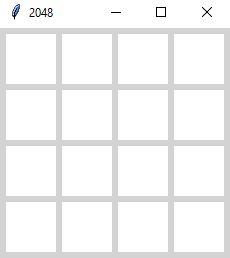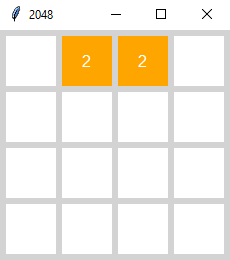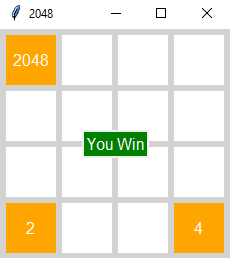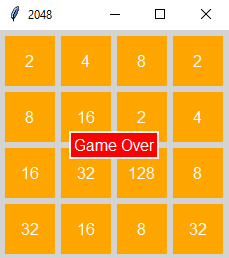×

Search anything:

# 2048 game in Python

#### PythonGet this book -> Problems on Array: For Interviews and Competitive Programming

2048 game is a fun and addictive single-player game that keeps you hooked from the moment you start playing. In this article, we will explain the strategies of the game and implement the steps in code using Python.

# How to play

This is a tile-sliding game. It means, it has is a board-game which has numbers on tiles. We slide the tiles and merge the numbers. We can only slide the tiles to Up, Down, Left, and Right directions. Choosing a direction will mean every element on the board will move to that direction.

Only two same numbers will merge; for example, we can merge 2 and 2, but we cannot merge 2 and 4. The goal is to merge the numbers until we get 2048. Merging numbers to 2048 will mean we have won the game. Otherwise, if there are no empty tiles left and we cannot merge any more numbers, the game is over and we lose.

At the beginning of the game, two 2's are randomly generated to start the game. As we swipe from left to right or any other direction, with every move a random number 2 or 4 is added to board. This addition of random number happens whether the other tiles are merged or not.

# Implementation

We will be explaining the steps as we implement the code. For this game, we have chosen a 4X4 matrix; that would be a square board with 4 rows and 4 columns.

To show the board and get the similar experience of actually playing the game, we are using the standard GUI library Tkinter for the graphical interface. It provides widgets that can be easily implemented as needed. We are also importing the random library to generate random numbers.

"Frame" widget in Tkinter keeps other widgets organized and working together. The colors for the board and the tiles are randomly picked and methods are defined for every function of the game.

# Start the Code

We are using a Game class so that all the functions and variables needed to build the game can be used easily. We are using the title "2048" for the game. Frame is used for keeping the functions grouped and well-maintained.

``````from tkinter import *
import random

class Game(Frame):
def __init__(self):
Frame.__init__(self)
self.grid()
self.master.title("2048")
self.main_grid = Frame(self, bg='lightgrey', bd=3, width=400,height=400)
self.main_grid.grid()
``````

# Make the Board

Now we create the 4X4 Gameboard using nested lists.

``````    def gameboard(self):
self.tiles = []
for i in range(4):
row = []
for j in range(4):
tile_frame = Frame(
self.main_grid,
bg='white',
width='50',
height='50'
)
tile_frame.grid(
row=i, column=j,
)
tile_number = Label(
self.main_grid,
bg='white'
)
tile_number.grid(row=i, column=j)
tile_data =
{
"frame": tile_frame,
"number": tile_number
}
row.append(tile_data)
self.tiles.append(row)
``````

A "tiles" list is created for storing the tiles of the grid. Adding the grid to the frame and tile_number to the grid. Tile numbers are created using Label widget. Tile number refers to each and every tile on the board. The tile data refers to the values we are supposed to merge. Tile data is used as a dictionary to store the values of the tiles.

The board will look like this:# Start Game

At the start of the game, the board will contain two random 2's at two random positions.

• First, we declare a nested list for the board where all the values are 0.
• Then we loop through the board and use "random" library methods to find two random tiles that contains 0. This 0 value will mean the tile is empty and a value can be inserted.# Operations of The Game

To perform the merge towards up, down, left, or right, we need functions that can be used for merging the values. Instead of writing a huge number of different methods for each of the operations, we can write some basic functions that can be used by them all.

For that we are going to stack all the values to the left of the board for every operation.

• We create a new matrix
• Add the elements of the board to the matrix. The board elements will remain on the same row but move to the left-most column(if it's empty) of that row on the new matrix.
• After getting all the values to the left side, we assign the new matrix to the board.

MoveToLeft

``````    def moveToLeft(self):
new_board = [[0 for col in range(4)] for row in range(4)]
for i in range(4):
fill_position = 0
for j in range(4):
if self.board[i][j] != 0:
new_board[i][fill_position] = self.board[i][j]
fill_position += 1
self.board = new_board
``````

For the merge function, first we check if the value is not 0 and if it is same as the value next to it. Instead of adding the values we simply multiply the value with 2 and we set the tile next to it to 0.

Merge

``````    def merge(self):
for i in range(4):
for j in range(3):
if self.board[i][j] != 0 and self.board[i][j] == self.board[i][j+1]:
self.board[i][j] *= 2
self.board[i][j+1] = 0
``````

Then we create a reverse function that will reverse the rows of the board. We declare a new matrix to store the reverse rows and then set the board to the new matrix.

Reverse

``````    def reverse(self):
new_board = []
for i in range(4):
new_board.append([])
for j in range(4):
new_board[i].append(self.board[i][3-j])
self.board = new_board
``````

The last function is the transpose function. This function changes the values diagonally. It means the values of board and board will swap.

Transpose

``````    def transpose(self):
new_board = [[0 for col in range(4)] for row in range(4)]
for i in range(4):
for j in range(4):
new_board[i][j] = self.board[j][i]
self.board = new_board
``````

# Pick Random Value

After each move, a random number(2 or 4) will be added to a random tile. For this, we are declaring a function that randomly generates row and column number for a random tile that is empty and available for inserting a value.

``````    def pickNewValue(self):
row = col = 0
while self.board[row][col] != 0:
row = random.randint(0, 3)
col = random.randint(0, 3)

if random.randint(1, 5) == 1:
self.board[row][col] = 4
else:
self.board[row][col] = 2
``````

# Update The Board

After every move, we need to update the board to see the result of that move. After every move, we are updating the values of the tiles and inserting a random 2 or 4 on a random tile. We are using tile_data dictionary to store tile value.

``````    def updateGame(self):
for i in range(4):
for j in range(4):
tile_value = self.board[i][j]
if tile_value == 0:
self.tiles[i][j]["frame"].configure(bg="white")
self.tiles[i][j]["number"].configure(bg="white", text="")
else:
self.tiles[i][j]["frame"].configure(bg="orange")
self.tiles[i][j]["number"].configure(
bg="orange",
fg="white",
font="20",
text=str(tile_value)
)
``````

# Moves For The Game

Now, we write the functions of Left, Right, Up, and Down.

Left

To merge the elements to the left, we need to take them all to the left and merge. Then we add a random 2 or 4 and we update the board.

``````    def left(self, event):
self.moveToLeft()
self.merge()
self.moveToLeft()
self.pickNewValue()
self.updateGame()
self.final_result()
``````

Right

The move to the right will reverse the rows, move the elements to the left, merge, move them again to the left, and reverse the rows back. Then place a random 2 or 4 and update the board.

``````    def right(self, event):
self.reverse()
self.moveToLeft()
self.merge()
self.moveToLeft()
self.reverse()
self.pickNewValue()
self.updateGame()
self.final_result()
``````

Up

This move requires the elements to change diagonally first. Then we move them to the left, merge the values, move to the left again to eliminate the 0 created in the empty space, and transpose the values back. Then we repeat the process of picking a random value and updating the game.

``````    def up(self, event):
self.transpose()
self.moveToLeft()
self.merge()
self.moveToLeft()
self.transpose()
self.pickNewValue()
self.updateGame()
self.final_result()
``````

Down

In this move, we mix all of the steps takes for previous moves. For this, we first need to transpose, then reverse, move values to the left, merge, move them back ti left, reverse back, and transpose again. Then the process of picking random value and updating game will repeat.

``````   def down(self, event):
self.transpose()
self.reverse()
self.moveToLeft()
self.merge()
self.moveToLeft()
self.reverse()
self.transpose()
self.pickNewValue()
self.updateGame()
self.final_result()
``````

# Check For Moves

If the board fills up with values we need to check if any two values can be merged. In other words, if any move is left. We create a function that checks if we can apply any move up or down, and left or right.

``````    def horizontal_move_exists(self):
for i in range(4):
for j in range(3):
if self.board[i][j] == self.board[i][j+1]:
return True
return False

def vertical_move_exists(self):
for i in range(3):
for j in range(4):
if self.board[i][j] == self.board[i+1][j]:
return True
return False
``````

# End of The Game

The game ends in two cases.

1. We find 2048, which means the game is over and we win
2. The board is filled up with values and no move left, which means the game is over and we lose.

We write a function to check for both cases. In the winning case the board will show a green sign with "You Win" writing and for the opposite case, the sign will be red with "Game Over" writing. The function for final result is called after updating the board in every move.

``````    def final_result(self):
if any(2048 in row for row in self.board):
game_over_frame = Frame(self.main_grid, borderwidth=2)
game_over_frame.place(relx=0.5, rely=0.5, anchor='center')
Label(
game_over_frame,
text="You Win",
bg="green",
fg="white",
font="20"
).pack()

elif not any(0 in row for row in self.board) and not self.horizontal_move_exists() and not self.vertical_move_exists():
game_over_frame = Frame(self.main_grid, borderwidth=2)
game_over_frame.place(relx=0.5, rely=0.5, anchor='center')
Label(
game_over_frame,
text="Game Over",
bg="Red",
fg="white",
font="20"
).pack()
``````

# Show Output

To show the gameboard and all the graphics we need to add some more steps. First, we need to call the functions to create the board and start the game in the init method at the beginning of the code. There we also need to bind the moves to keyboard press. The mainloop() is added to keep the GUI running.

``````class Game(Frame):
def __init__(self):
Frame.__init__(self)
self.grid()
self.master.title("2048")
self.main_grid = Frame(self, bg='lightgrey', bd=3, width=400,height=400)
self.main_grid.grid()

#call the functions to run the program
self.gameboard()
self.start_game()

self.master.bind("<Left>", self.left)
self.master.bind("<Right>", self.right)
self.master.bind("<Up>", self.up)
self.master.bind("<Down>", self.down)

self.mainloop()
``````

At the end of the code, we need to create an instance of the class Game to run the code.

``````def main():
Game()

if __name__ == "__main__":
main()
``````

# Final Results

For winning case:For losing case:With this article at OpenGenus, you must have the complete idea of how to develop 2048 game in Python as a project and boost your Software Developer Portfolio.#### Muhsina Munfa

Muhsina Munfa is a graduate with a BSc degree in the Department of CSE from Metropolitan University Sylhet.